Question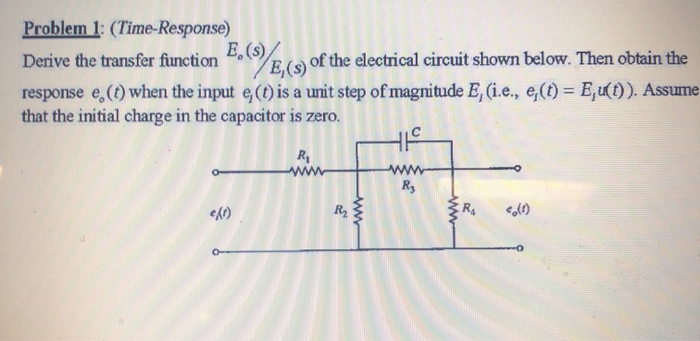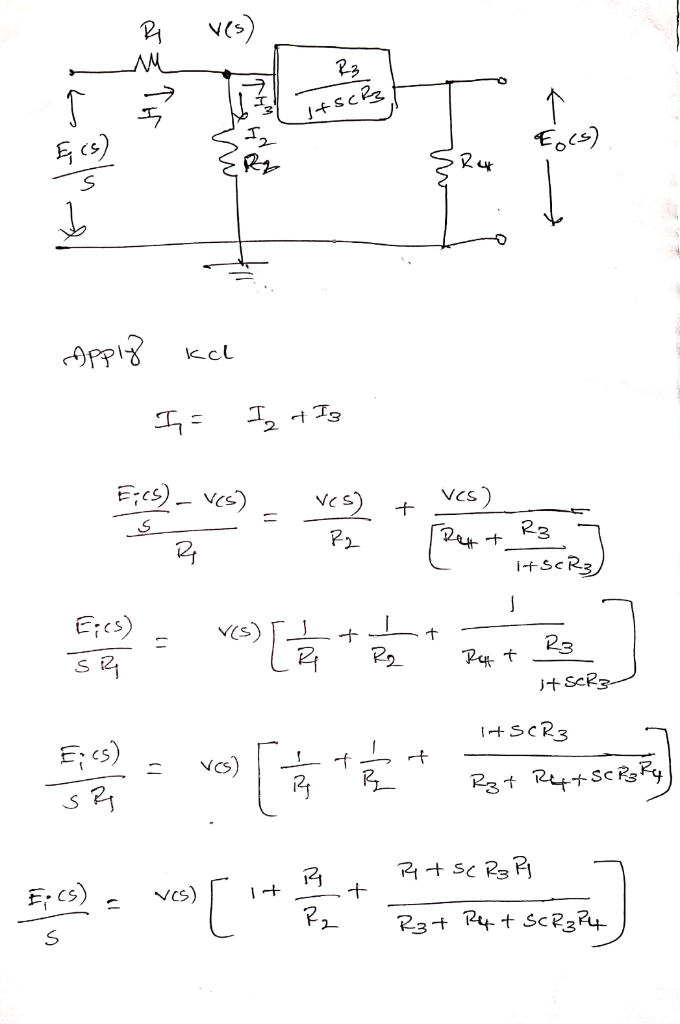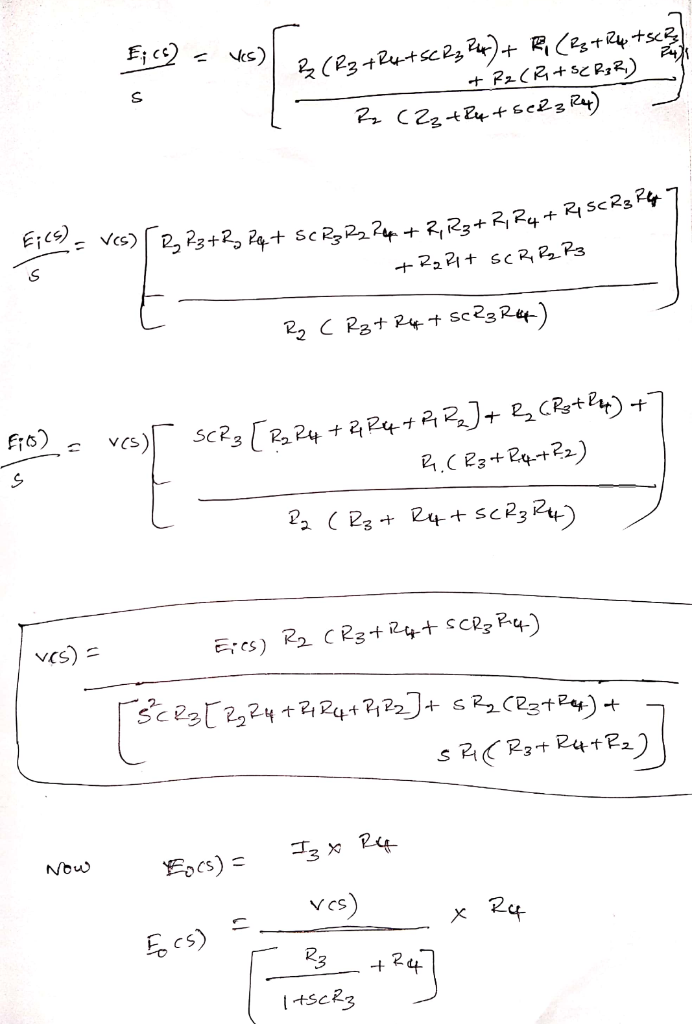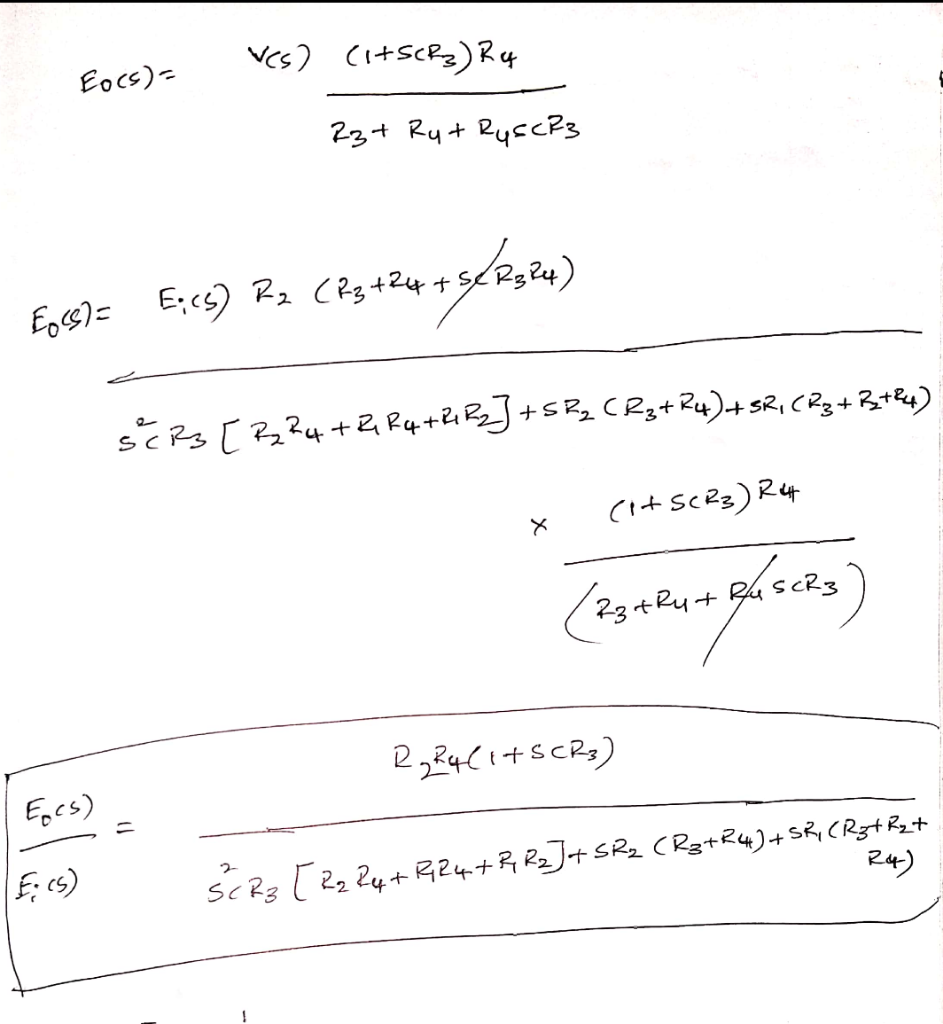#### Earn Coins

Coins can be redeemed for fabulous gifts.

Similar Homework Help Questions
• ### thx!!!! Question 3 (5.5 marks) a) Find the transfer function of the electrical circuit shown in...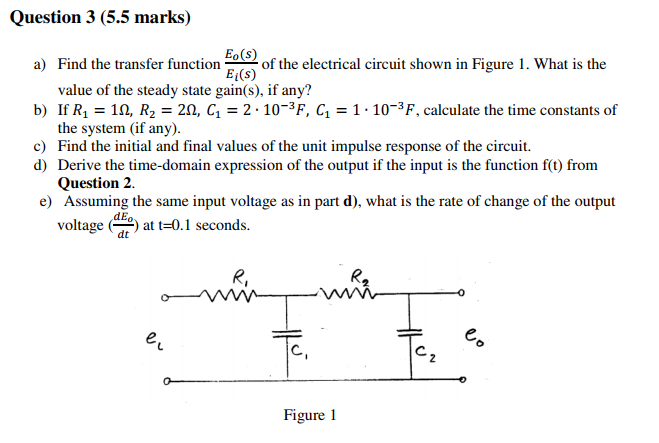thx!!!! Question 3 (5.5 marks) a) Find the transfer function of the electrical circuit shown in Figure 1. What is the value of the steady state gain(s), if any? b) If R1 1, R2 = 2n, C\ = 2- 10-3F, C 1-10-3F, calculate the time constants of the system (if any). c) Find the initial and final values of the unit impulse response of the circuit d) Derive the time-domain expression of the output if the input is the function...

• ### Q11 (20pts.): For the circuit shown below Derive the transfer function, H(s) assuming the OPAMP's transfer...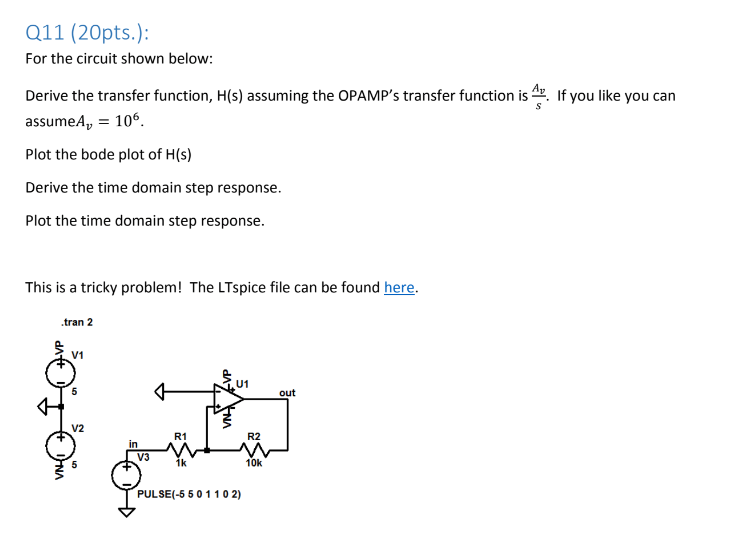Q11 (20pts.): For the circuit shown below Derive the transfer function, H(s) assuming the OPAMP's transfer function is. If you like you can assumeA 106 Plot the bode plot of H(s) Derive the time domain step response. Plot the time domain step response This is a tricky problem! The LTspice file can be found here. .tran 2 V1 U1 out V2 R1 R2 in V3 1k 10k PULSE(-5 50 1102)

• ### 2. (14 marks total) This question deals with the series RLC circuit discussed in the classroom...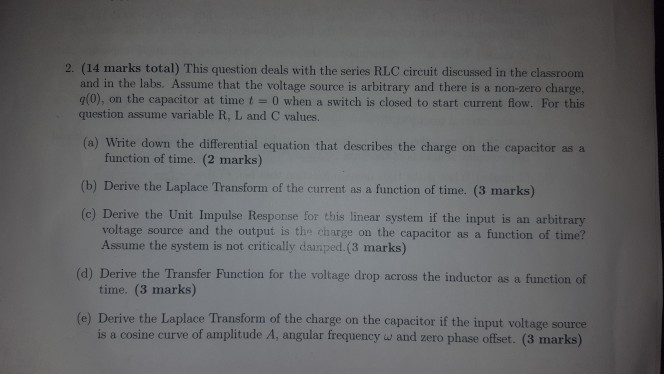2. (14 marks total) This question deals with the series RLC circuit discussed in the classroom and in the labs. Assume that the voltage source is arbitrary and there is a non-zero charge, g(0), on the capacitor at time t 0 when a switch is closed to start current flow. For this question assume variable R, L and C values. (a) Write down the differential equation that describes the charge on the capacitor as a function of time. (2 marks)...

• ### System Modeling and Laplace transform: In this problem we will review the modeling proce- dure for the RLC circuit as shown below, and how to find the corresponding transfer function and step respons...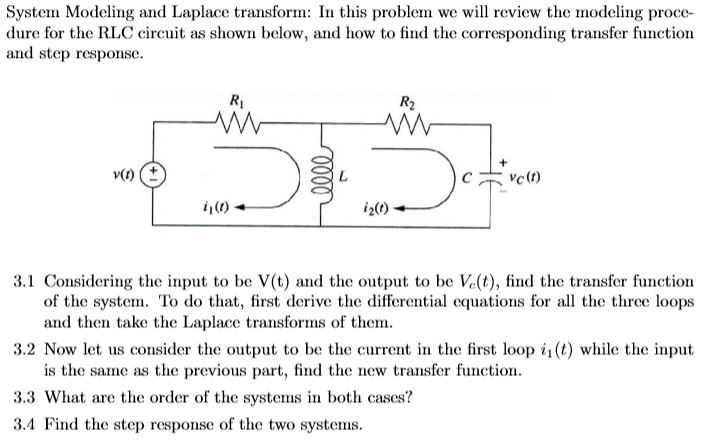System Modeling and Laplace transform: In this problem we will review the modeling proce- dure for the RLC circuit as shown below, and how to find the corresponding transfer function and step response Ri R2 Cv0) i2) i,(0) 3.1 Considering the input to be V(t) and the output to be Ve(t), find the transfer function of the system. To do that, first derive the differential equations for al the three loops and then take the Laplace transforms of them. 3.2...

• ### Consider the automobile cruise-control system shown below: Engine ActuatorCarburetor 0.833 and load 40 3s +1 Compensator R(s)E(s) Ge(s) s +1 -t e(t) Sensor 0.03 1) Derive the closed-loop transfer fun...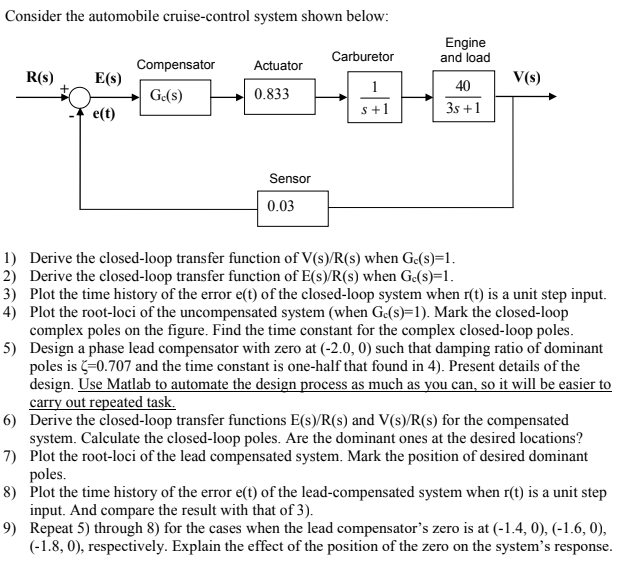Consider the automobile cruise-control system shown below: Engine ActuatorCarburetor 0.833 and load 40 3s +1 Compensator R(s)E(s) Ge(s) s +1 -t e(t) Sensor 0.03 1) Derive the closed-loop transfer function of V(s)/R(s) when Gc(s)-1 2) Derive the closed-loop transfer function of E(s)/R(s) when Ge(s)-1 3) Plot the time history of the error e(t) of the closed-loop system when r(t) is a unit step input. 4) Plot the root-loci of the uncompensated system (when Gc(s)-1). Mark the closed-loop complex poles on...

• ### Find the ratio Vo(s)/Vi(s) for the electrical network shown in the figure below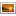Q1.png 1. Find the ratio Vds)/(s) for the electrical network shown in Fig. 5 1,6) R. V(+) C2 V. (D) b) For the transfer function in 5b, find the time response if m=1, B=3 and K=2 to an input Viſt)= 5u(t), where u(t) is unit step function

• ### 1. Consider the circuit shown below. Cl e, (0) c, e。(t) Find the transfer function below using t...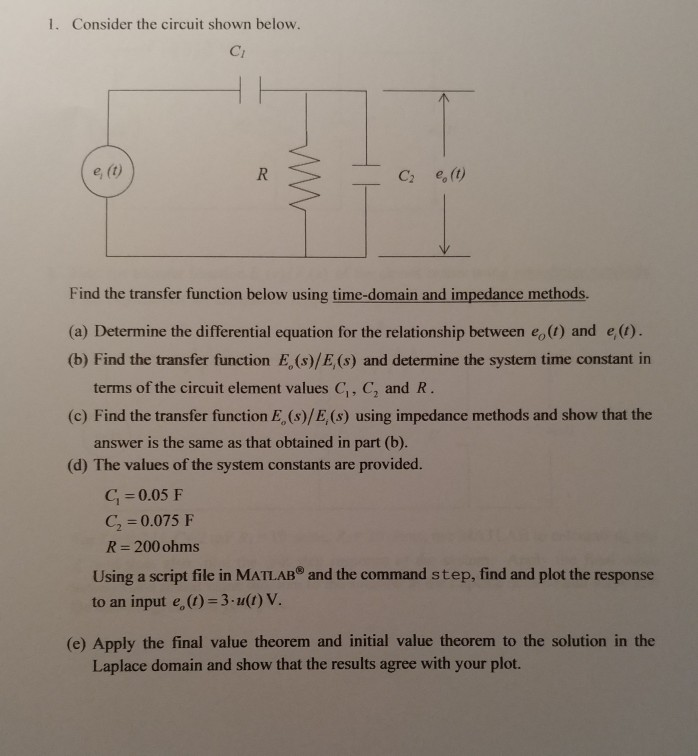Please help with this dynamics circuit analysis. Please show work and explain. Thank you!! 1. Consider the circuit shown below. Cl e, (0) c, e。(t) Find the transfer function below using time-domain and impedance methods. (a) Determine the differential equation for the relationship between eo(1) and e(1) (b) Find the transfer function E, (s)/E,(s) and determine the system time constant in terms of the circuit element values C, C, and R 17 2 (c) Find the transfer function E, (s)/E,...

• ### Question # 1 : Find the transfer function represented by: ??(?) ?? + 5 ?(?) =...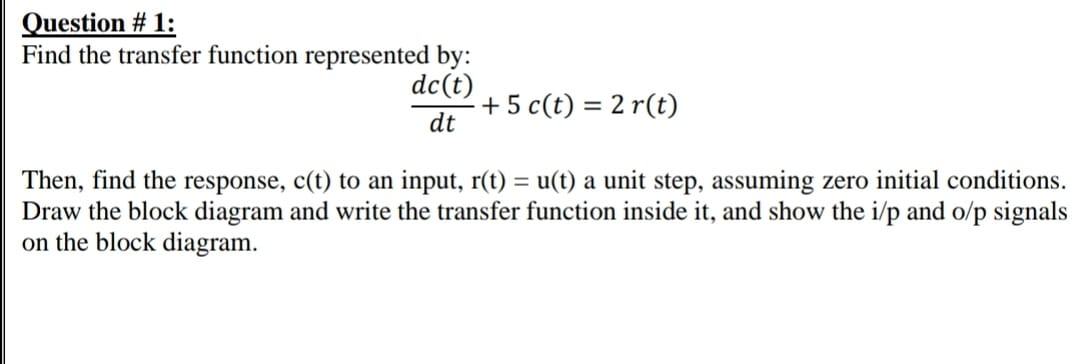Question # 1 : Find the transfer function represented by: ??(?) ?? + 5 ?(?) = 2 ?(?) Then, find the response, c(t) to an input, r(t) = u(t) a unit step, assuming zero initial conditions. Draw the block diagram and write the transfer function inside it, and show the i/p and o/p signals on the block diagram. Question #1: Find the transfer function represented by: dc(t) + 5 c(t) = 2 r(t) dt Then, find the response, c(t) to...

• ### Electrical engineering problem 1251 Problem 2 For the circuit below a) Convert input V.lt) into Amplitude and phase form b) Find the output response V(t) using Fourier series in the circuit shown, dra...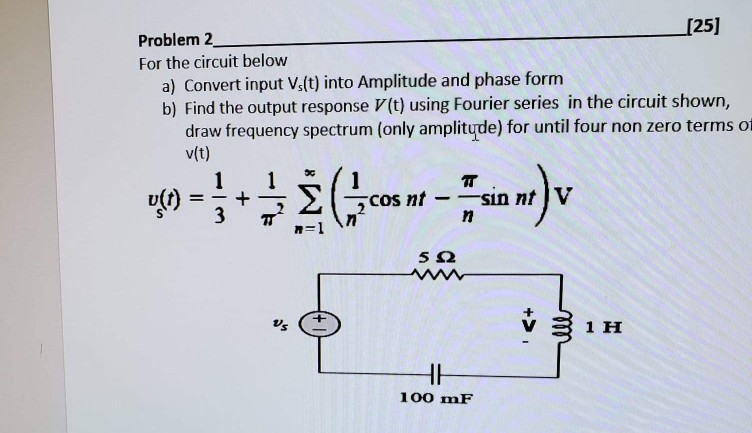Electrical engineering problem 1251 Problem 2 For the circuit below a) Convert input V.lt) into Amplitude and phase form b) Find the output response V(t) using Fourier series in the circuit shown, draw frequency spectrum (only amplityde) for until four non zero terms o v(t) 5Ω 1 H 100 mF 1251 Problem 2 For the circuit below a) Convert input V.lt) into Amplitude and phase form b) Find the output response V(t) using Fourier series in the circuit shown, draw...

• ### Problem B-4-1 Find the transfer function X (s)/X/(s) of the mechanical system shown in Figure 4-50....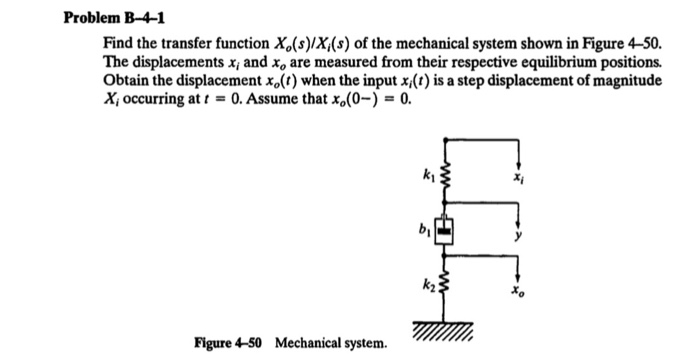Problem B-4-1 Find the transfer function X (s)/X/(s) of the mechanical system shown in Figure 4-50. The displacements x; and X, are measured from their respective equilibrium positions. Obtain the displacement xo(t) when the input x;(t) is a step displacement of magnitude X; occurring at t = 0. Assume that x.(0-) = 0. T Figure 4-50 Mechanical system.